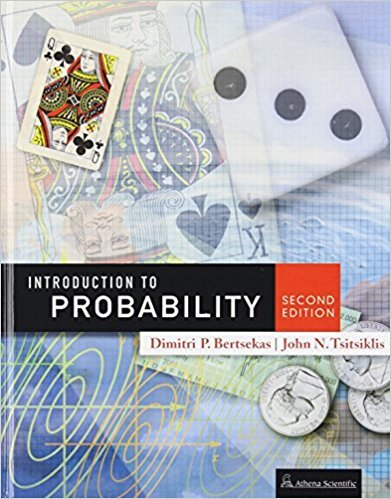×
×

# Solutions for Chapter 1: Sample Space and Probability## Full solutions for Introduction to Probability, | 2nd Edition

ISBN: 9781886529236Solutions for Chapter 1: Sample Space and Probability

Solutions for Chapter 1
4 5 0 339 Reviews
12
2
##### ISBN: 9781886529236

Since 62 problems in chapter 1: Sample Space and Probability have been answered, more than 7362 students have viewed full step-by-step solutions from this chapter. Chapter 1: Sample Space and Probability includes 62 full step-by-step solutions. Introduction to Probability, was written by and is associated to the ISBN: 9781886529236. This textbook survival guide was created for the textbook: Introduction to Probability,, edition: 2. This expansive textbook survival guide covers the following chapters and their solutions.

Key Statistics Terms and definitions covered in this textbook
• 2 k p - factorial experiment

A fractional factorial experiment with k factors tested in a 2 ? p fraction with all factors tested at only two levels (settings) each

• Bayes’ theorem

An equation for a conditional probability such as PA B ( | ) in terms of the reverse conditional probability PB A ( | ).

• Central tendency

The tendency of data to cluster around some value. Central tendency is usually expressed by a measure of location such as the mean, median, or mode.

• Coeficient of determination

See R 2 .

• Comparative experiment

An experiment in which the treatments (experimental conditions) that are to be studied are included in the experiment. The data from the experiment are used to evaluate the treatments.

• Conditional probability density function

The probability density function of the conditional probability distribution of a continuous random variable.

• Continuity correction.

A correction factor used to improve the approximation to binomial probabilities from a normal distribution.

• Control chart

A graphical display used to monitor a process. It usually consists of a horizontal center line corresponding to the in-control value of the parameter that is being monitored and lower and upper control limits. The control limits are determined by statistical criteria and are not arbitrary, nor are they related to speciication limits. If sample points fall within the control limits, the process is said to be in-control, or free from assignable causes. Points beyond the control limits indicate an out-of-control process; that is, assignable causes are likely present. This signals the need to ind and remove the assignable causes.

• Convolution

A method to derive the probability density function of the sum of two independent random variables from an integral (or sum) of probability density (or mass) functions.

• Correction factor

A term used for the quantity ( / )( ) 1 1 2 n xi i n ? = that is subtracted from xi i n 2 ? =1 to give the corrected sum of squares deined as (/ ) ( ) 1 1 2 n xx i x i n ? = i ? . The correction factor can also be written as nx 2 .

• Correlation matrix

A square matrix that contains the correlations among a set of random variables, say, XX X 1 2 k , ,…, . The main diagonal elements of the matrix are unity and the off-diagonal elements rij are the correlations between Xi and Xj .

• Cumulative distribution function

For a random variable X, the function of X deined as PX x ( ) ? that is used to specify the probability distribution.

• Density function

Another name for a probability density function

• Expected value

The expected value of a random variable X is its long-term average or mean value. In the continuous case, the expected value of X is E X xf x dx ( ) = ?? ( ) ? ? where f ( ) x is the density function of the random variable X.

• Exponential random variable

A series of tests in which changes are made to the system under study

• Factorial experiment

A type of experimental design in which every level of one factor is tested in combination with every level of another factor. In general, in a factorial experiment, all possible combinations of factor levels are tested.

• First-order model

A model that contains only irstorder terms. For example, the irst-order response surface model in two variables is y xx = + ?? ? ? 0 11 2 2 + + . A irst-order model is also called a main effects model

• Fisher’s least signiicant difference (LSD) method

A series of pair-wise hypothesis tests of treatment means in an experiment to determine which means differ.

• Fixed factor (or fixed effect).

In analysis of variance, a factor or effect is considered ixed if all the levels of interest for that factor are included in the experiment. Conclusions are then valid about this set of levels only, although when the factor is quantitative, it is customary to it a model to the data for interpolating between these levels.

• Harmonic mean

The harmonic mean of a set of data values is the reciprocal of the arithmetic mean of the reciprocals of the data values; that is, h n x i n i = ? ? ? ? ? = ? ? 1 1 1 1 g .

×